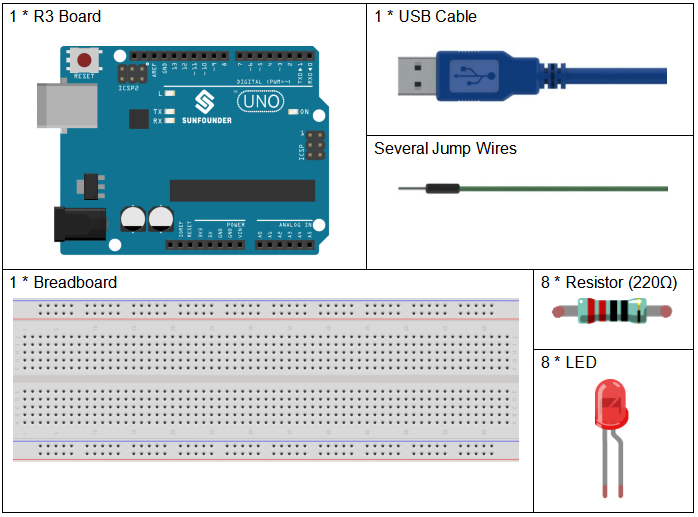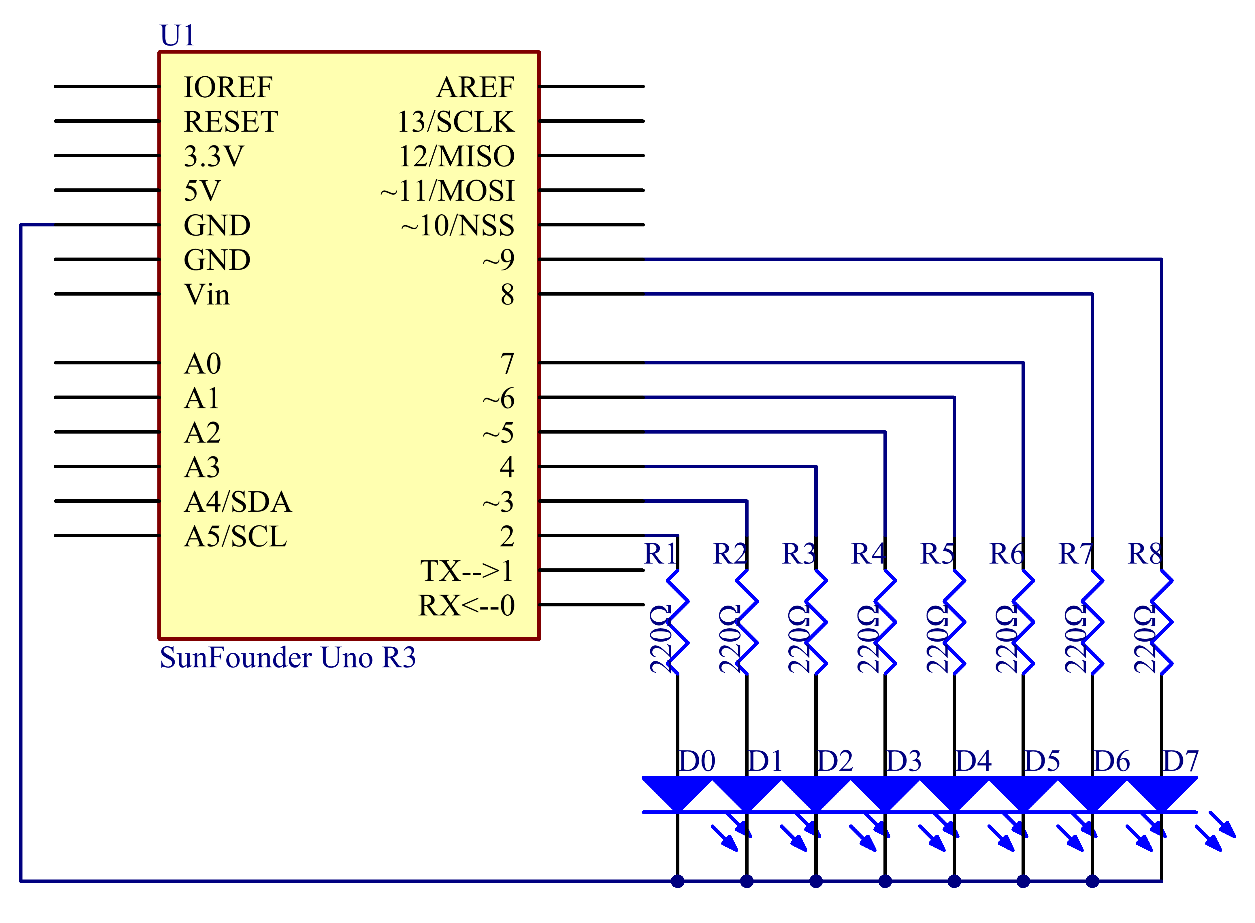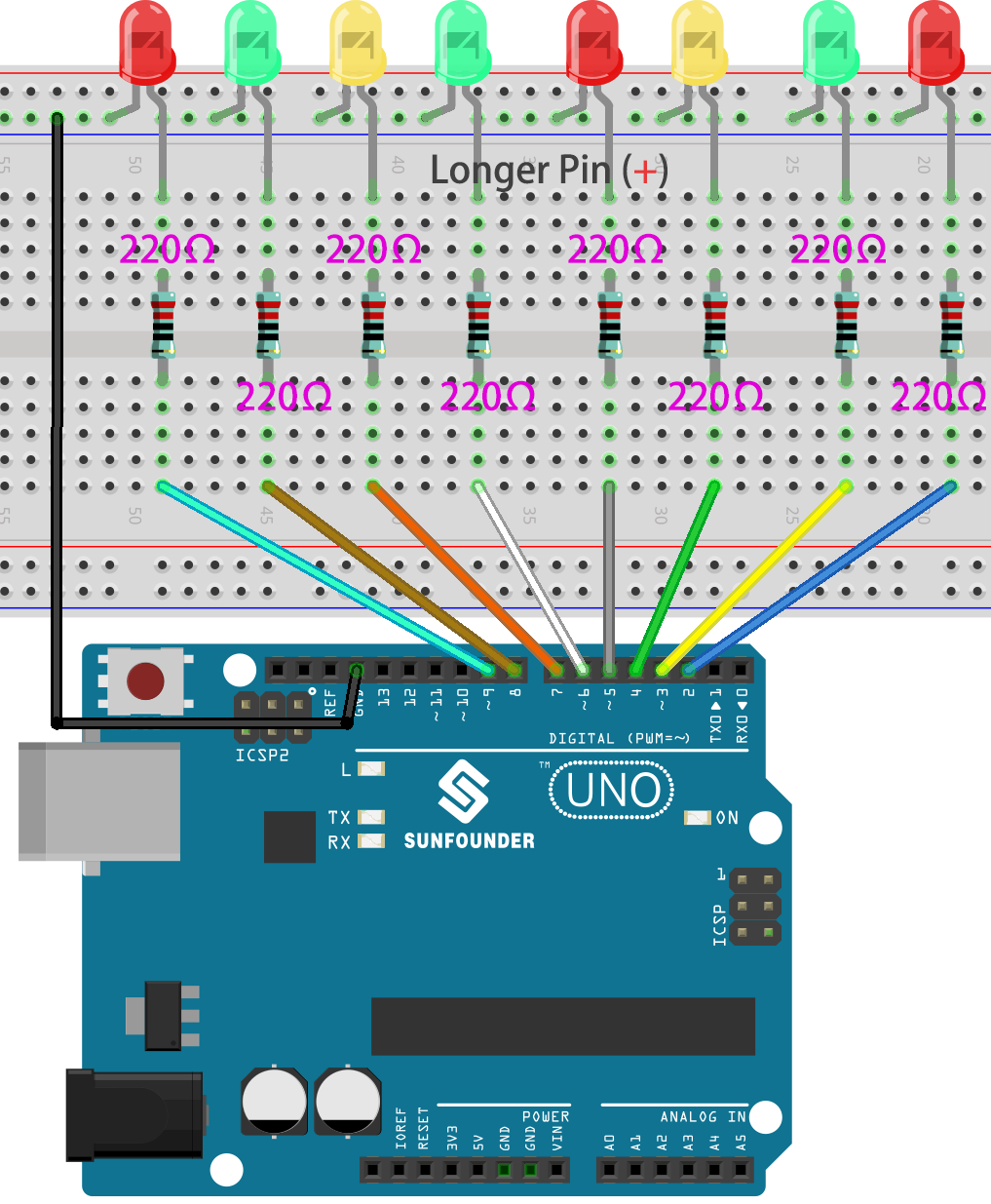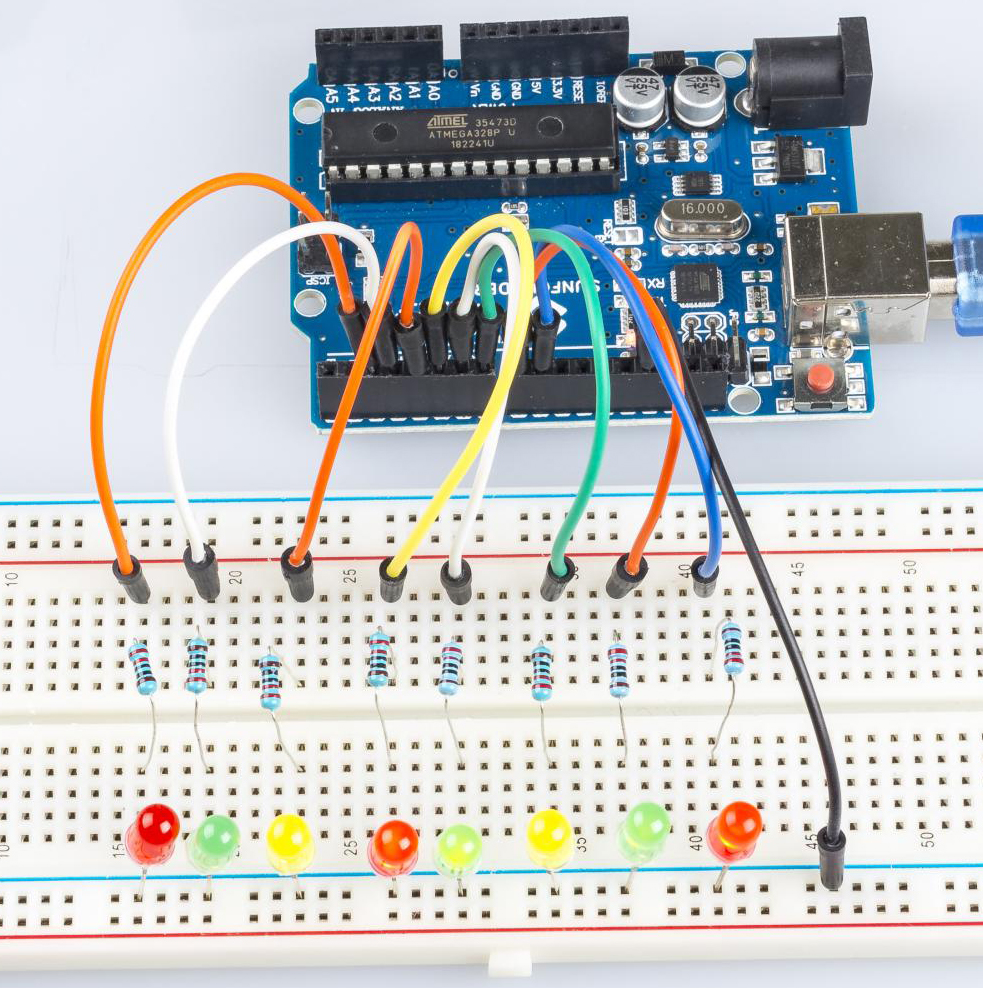# Lesson 2 Flowing LED Lights¶

## Introduction¶

In this lesson, we will conduct a simple yet interesting experiment – using LEDs to create flowing LED lights. As the name suggests, these eight LEDs in a row successively light up and dim one after another, just like flowing water.

## Components¶## Schematic Diagram¶

The principle of this experiment is simply to turn on eight LEDs in turn. The eight LEDs are connected to pin 2-pin 9 respectively. Set them as High level and the corresponding LED at the pins will light up. Control the time of each LED brightening and you will see flowing LED lights.## Experimental Procedures¶

Step 1: Build the circuit.

Step 2: Open the code file.

Step 3: Select the Board and Port.

Step 4: Upload the sketch to the board.Now, you should see eight LEDs brighten one by one from the LED connected to pin 2 to that to pin 9, and then dim in turn from the LED at pin 9 to the one at pin 2. After that, the LEDs will light up from the LED at pin 9 to that at pin 2 and dim from the LED at pin 2 to that at pin 9. This whole process will repeat until the circuit is power off.## Code Analysis¶

for() statement

```for (int i = 2; i <= 9; i++)
/*8 LEDs are connect to pin2-pin9, When i=2,
which accords with the condition i<=9,
then run the code in the curly braces, set the pin2 to OUTPUT.
After that run i++(here in i = i + 1, the two "i"s are not the same,
but i\ :sub:`now` = i\ :sub:`before` + 1).
Use the for() statement to set pin 2-pin 9 as output respectively.*/

{

pinMode(i, OUTPUT); //initialize a as an output

}
```

for (initialization; condition; increment) { //statement(s); }: The for statement is used to repeat a block of statements enclosed in curly braces.The initialization happens first and exactly once. Each time through the loop, the condition is tested; if it’s true, the statement block, and the increment is executed, then the condition is tested again. When the condition becomes false, the loop ends.

Set flowing led lights

Use the for() statement to set pin2-pin9 to a high level inturn.

```for (int a = 2; a <= 9; a++)

{

digitalWrite(a, HIGH); //turn this led on

delay(100); //wait for 100 ms

}
```

Then let the 8 LEDs go out from pin9 to pin2 in turn.

```for (int a = 9; a <= 2; a--)

{

digitalWrite(a, LOW); //turn this led on

delay(100); //wait for 100 ms

}
```

Finally, use the same way to turn on the 8 LEDs from pin9 to pin2 in turn and let them go out in turn.

```for (int a = 9; a <= 2; a--)

{

digitalWrite(a, HIGH); //turn this led on

delay(100); //wait for 100 ms

}

for (int a = 2; a <= 9; a++)

{

digitalWrite(a, LOW); //turn this led on

delay(100); //wait for 100 ms

}
```

Experiment Summary

Through this experiment, you have learned how to use for() statement which is a very useful statement when you want to short the code.